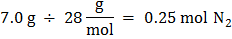### Sample Problem

Consider the following balanced equation:

N2 + 3H2 → 2NH3

How many grams of NH3 are produced if 7.0 grams of N2 react? (Molar mass of NH3 = 17 g/mol, molar mass of N2 = 28 g/mol)

g

#### Solution0.25 mol N2  x 2 = 0.50 mol NH3

0.50 mol NH3 x 17 g/mol =8.5 g